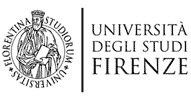Salta gli elementi di navigazioneDIMAI Dipartimento di Matematica e Informatica 'Ulisse Dini'
Menù principale

# Analisi Matematica

## Research topics

### Calculus of variations

• Regularity of operators with anisotropic and general growth
• Semicontinuity in the Calculus of Variations
• Fractional Operators

Vespri

• Density functional theory for Coulomb or interacting systems

De Pascale

• Optimal transport problems and applications

De Pascale

• Variational solutions to evolutionary problems
• Free discontinuity problems Free boundary problems

### Convex analysis

• Theory of convex bodies
• Geometric tomography
• Geometric inequalities

### Dynamical systems

• Non-autonomous dynamical systems and ergodic theory

Fabbri

• PDE’s and infinite dimensional dynamical systems: long-time behavior, attractors

### Mathematical Control Theory

• Optimal Control, Hamiltonian methods, Geometric control
• Control of Partial Differential Equations (PDE’s) and Infinite Dimensional Systems
• Nonautonomous linear control systems

Fabbri

### Partial differential equations

• Applications to mathematical physics
• Geometric and functional inequalities
• Qualitative properties of solutions
• Quantitative estimates of unique continuation
• Regularity estimates

### Ordinary differential equations

• Qualitative theory for ordinary differential equations and inclusions
• Boundary value problems on compact and noncompact intervals
• Functional differential and differential-difference equations and inclusions
• Topological methods for ordinary differential equations and inclusions
• Non-autonomous dynamics: non-autonomous dynamical systems and ergodic theory
• Topological dynamics: Lypaunov exponents, exponential dychotomies and rotation numbers
• Non autonomous Hamiltonian systems, quasi-periodic Schroedinger operators
• Bifurcation theory

## Postdocs

• Luca Bisconti
• Marco Caroccia
• Giovanni Franzina
• Francesco Geraci
• Alberto Roncoroni

## Other frequent collaborators

• P. Acquistapace
• A. Agrachev
• P. Benevieri
• E. Beretta
• V. Bögelein
• K. Boroczky
• G. Bouchitté
• G. Buttazzo
• A. Calamai
• G. Carlier
• T. Champion
• R. Chiappinelli
• F. C. Chittaro
• A. Constantin
• S. Conti
• G. Cupini
• B. Dacorogna
• C. De Lellis
• G. De Philippis
• E. DiBenedetto
• M. Di Cristo
• S. DiPierro
• F. Dragoni
• Z. Došlá
• F. Duzaar
• G. Duzgun
• M. Eleuteri
• M. Furi
• N. Fusco
• R. Gardner
• M.S. Gelli
• U. Gianazza
• G. Grillo
• M. Guerra
• K. Ishige
• F. Iurlano
• A. Henrot
• D. Henry
• M. Ishiwata
• M. Ludwig
• F. Leonetti
• M. Marini
• J. Mawhin
• A. Morassi
• S. Novo
• C. Nunez
• R. Obaya
• V. Obukhovskii
• D. Papini
• P. Réhâk
• E. Rosset
• S. Sakaguchi
• F. Santambrogio
• A. Slavova
• G. Stefani
• A. Takatsu
• E. Valdinoci
• F. Zanolin
• P. Zezza

## PhD Courses

ultimo aggiornamento: 04-Giu-2020